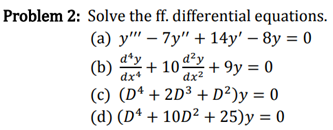### Create an Account

Already have account?

### Forgot Your Password ?

Home / Questions / Problem 2: Solve the ff. differential equations. (a) y'' – 7y" + 14y' - 8y = 0 d'y (b) dx2...

# Problem 2: Solve the ff. differential equations. (a) y'' – 7y" + 14y' - 8y = 0 d'y (b) dx2 +9y = 0 (C) (D4 + 2D3 + D2)y = 0 (d) (D4 + 10D2 +25)y = 0 +100% dx

Problem 2: Solve the ff. differential equations. (a) y'' – 7y" + 14y' - 8y = 0 d'y (b) dx2 +9y = 0 (C) (D4 + 2D3 + D2)y = 0 (d) (D4 + 10D2 +25)y = 0 +100% dxApr 13 2021 View more View Less

#### Answer (Solved)Subscribe To Get Solution